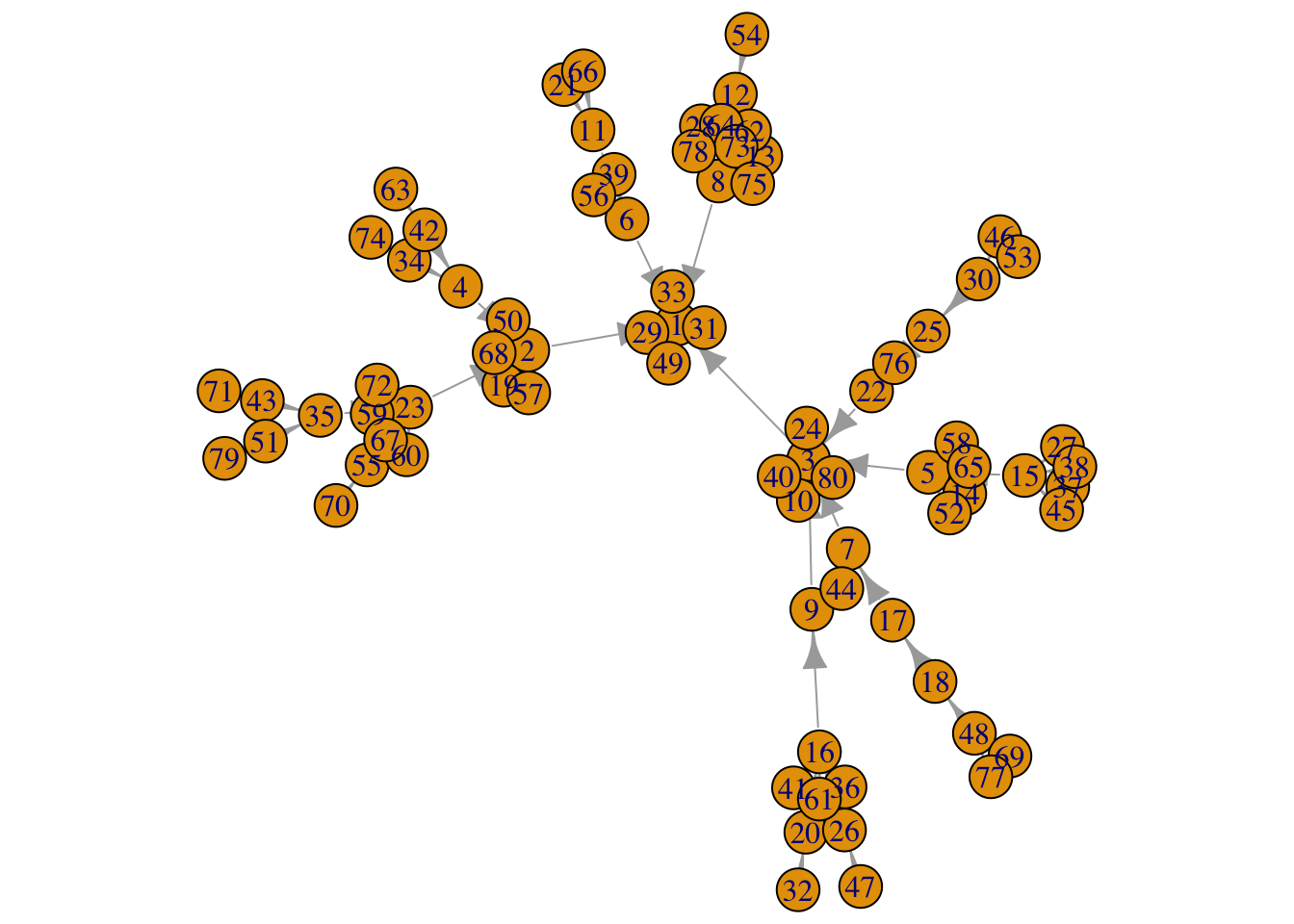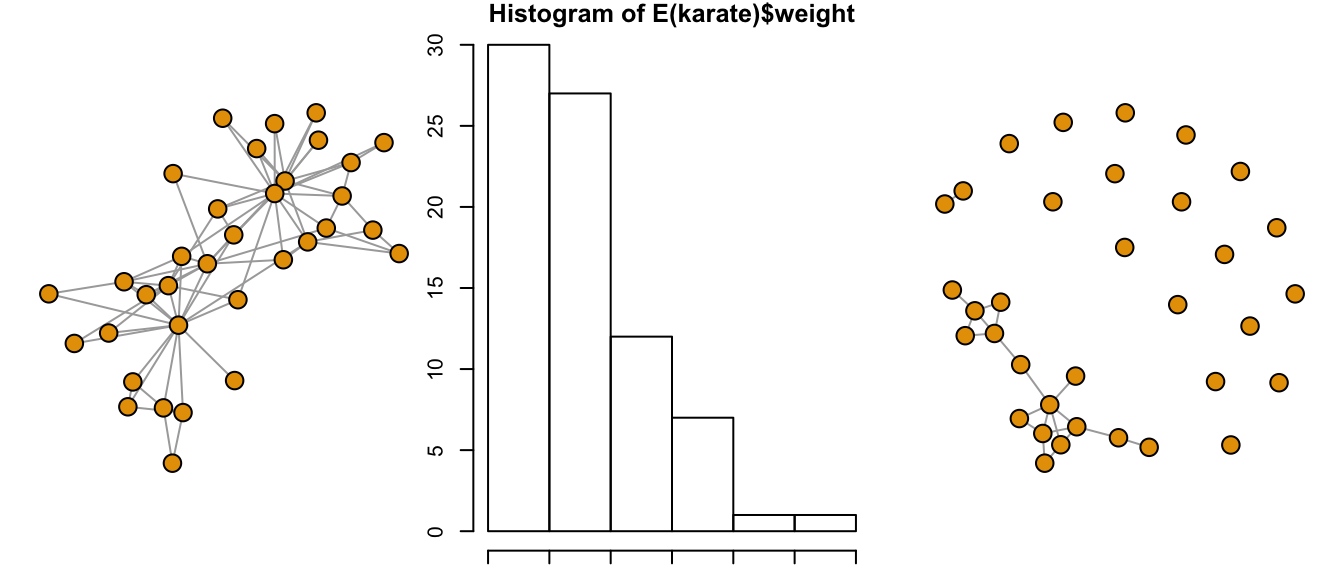# Chapter 5 Filter and Group

Important tasks in network analysis include omitting insignificant or nominal connections from a graph while highlighting and emphasizing others.

## 5.1 Pruning

Pruning is the process of eliminating insignifant or nominal connections from the network graph. There are many methods available and only the simplest of strategies are employed here. The example here is Zachary’s karate club, a resident dataset in the igraphdata package. More information may be found by entering ?igraphdata::karate.

g1 <- sample_pa(80)
l <- layout_with_fr(g1)
l <- norm_coords(l, ymin=-1, ymax=1, xmin=-1, xmax=1)
par(mar=c(0,0,0,0))
plot(g1, rescale=F, layout=l*1.0)data("karate")
karate.new <- delete_edges(karate, E(karate)[weight<=3])
par(mfrow = c(1, 3), mar = c(1,1,1,1))
plot(karate,
vertex.label = NA,
vertex.color = 1)
hist(E(karate)\$weight)
plot(karate.new,
vertex.label = NA,
vertex.color = 1)## 5.2 Grouping

Grouping is the process of associating nodes together via